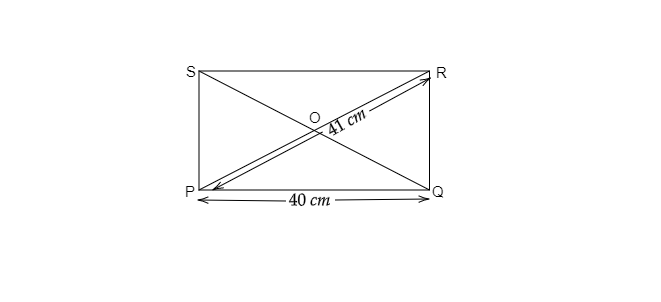# Find the perimeter of the rectangle whose length is $40\ cm$ and a diagonal is $41\ cm$.

Given: rectangle whose length is $40\ cm$ and a diagonal is $41\ cm$.

To do: To find the perimeter of the rectangle.

Solution:

$PR=41\ cm$, $PQ=40\ cm$Let breadth $(QR)$ be $x\ cm$

Now, in right-angled triangle PQR

$(PR)^2=(RQ)^2+(PQ)^2$

$\Rightarrow (41)^2=x^2+(40)^2$

$\Rightarrow 1681=x^2+1600$

$\Rightarrow 1681-1600=x^2$

$\Rightarrow \sqrt{81}=x$

$\Rightarrow 9=x$

Therefore, the breadth of the rectangle$=9\ cm$

The perimeter of the rectange$=2(l+b)$

$=2(40+9)$

$=2(49)$

$=98\ cm$.